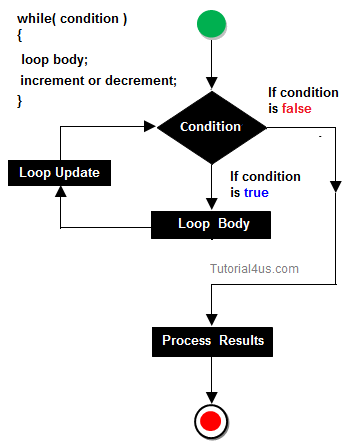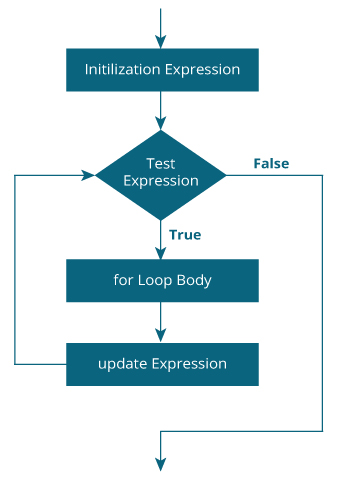#### JavaScript Loop and It's Example#### Loop definition

Loops in JavaScript are used to execute the same block of code a specified number of times or while a specified condition is true. Very often when you write code, you want the same block of code to run over and over again in a row.

The following are the looping statements in JavaScript:

#### i). While loop:

The most basic loop in JavaScript is the while loop. There are two key parts to a JavaScript while loop:

The conditional statement which must be true for the while loop's code to be executed.
The while loop's code that is contained in curly braces"{and}" will be executed if the condition is true.

When a while loop begins, the JavaScript interpreter checks if the condition statement is true. If it is, then the code between the curly braces is executed.

The same procedure is repeated until the condition stays true. If the condition statement is always True, then you will never exit the while loop.

#### Syntax:

```
while(expression)
{
Statement(s) to be executed if expression is tru
}

```

#### Flow Chart:```
sum=1275

```

```
loop = 0
loop = 1
loop = 2
loop = 3
loop = 4

```

#### ii). do-while loop

The do..while loop is similar to the while loop except that the condition check happens at the end of the loop.

This means that the loop will always be executed at least once, even if the condition is false.

#### Syntax:

```do
{
StatementI(s) to be executed;
}
while(expression);
```

#### Flow Chart```
loop = 0
loop = 1
loop = 2
loop = 3
loop = 4
loop = 5

```

#### iii). for loop

The for loop is the most compact form of looping and includes the following three important parts:

The loop initialization where we initialize our counter to a starting value. The initialization statement is executed before the loop begins.

The test statement which will test if the given condition is true or not. If condition is true then code given inside the loop will be execured otherwise loop will come out.

The iteration statement where counter value is incremented or decremented.

#### Syntax:

```
for(initialization; test condition; iteration statement)
{
Statements(s) to be executed if test condition is true
}

```

#### Flow Chart:```
Counter = 0
Counter = 1
Counter = 2
Counter = 3
```

#### Break Statements:

The break statement is used to exit a loop early. It breaks the execution of the code from that block.

```
break;

```

#### Continue Statement:

The continue statement tells the interpreter to immediately start the next iteration of the loop and skip remaining code block.

When a continue statement is encountered, program flow twill move to the loop check expression immediately and if condition remain true then it start next iteration otherwise control comes out of the loop.

#### Syntax:

```
continue;

```